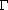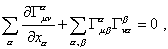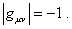V.5 No 2 5 On reality of black holes3. Schwarzschild's solution for the gravity field of point mass and its shortcomings To properly understand the difficulties that arise in solving the Schwarzschild's problem, it would be most reliable to analyse the whole derivation. The first discrepancy meets us at the start point: there exist several parallel derivations that lead to Schwarzschild's solution, and each in its own way juggles in order to come to the desirable result. This list of the statements of problem we will begin with the Schwarzschild's approach which he stated in his basic work "On the gravity field of point mass in Einsteinian theory" . Schwarzschild based his approach on the system of modelling equations which Einstein introduced in his calculation of motion of Mercury perihelion . This system is based on the following: "To these field equations correspond the generally covariant equations, if the scalar of the energy tensor of the 'matter' turns to zero, and it is established that no considerations of principle contradict to introduce this hypothesis, due to which the space and time lose their last sign of objective reality" [22, p. 439]. In connection with this Einstein's claim, all curtseys of Einstein and his followers towards the material space of gravitating masses lose their sense. With zero energy of space, it becomes again geometrically abstract and admits whatever manipulations with physical parameters convenient for relativists. None the less, let us take as the fact that "after Einstein's theory, these will be equations of a massless point in the gravity field of some mass located at the point x1 = x2 = x3 = 0 , if for the 'components of gravity field'anywhere except the point x1 = x2 = x3 = 0  the 'field equations'(12)
 as well as the 'condition for the determinant' (condition of zero energy of material space - Authors)(13)
 are true. The field equations with the condition for determinant have the basic property - they retain their appearance at the variables x1 ,  x2, x3 , x4 substituted by any other variables, if only the related Jacobian is equal to 1" [21, p. 200]. Let us stop and think, what does it mean from the physical view. The Jacobian equal to 1 says only, in geometrical sense the equation of motion (12) has to be invariant as to the reference frame transformation. But geometry only determines the metric - the laws that are true in such or other metric are determined by the physics of processes. The physics of processes evidences, in transition from inertial reference frames to non-inertial reference frames, the conservation laws and dynamic laws are fully violated. And relativists cannot state that for relativistic cases there will be one laws and for those classical - others. Firstly, with decreasing velocities, the relativistic laws have to reduce to the known laws of classical physics, as relativists say themselves. If in classical physics, at non-relativistic velocities, in non-inertial reference frames, the conservation laws are true, they will be true also in case of relativistic velocities. Secondly, Schwarzschild has formulated his problem for a stationary field and it does not premise in its statement any relativistic velocities of the reference frame. It premises the warping of space-time introduced by the gravity field, which even more imposes the ban, the motion equations to be conserved in transition from one non-inertial reference frame to another. Schwarzschild encountered it immediately when wrote down the metric describing the gravity field of a point mass.

Contents: / 1 / 2 / 3 / 4 / 5 / 6 / 7 / 8 / 9 / 10 / 11 / 12 / 13 / 14 / 15 / 16 / 17 /